• 共回答了15个问题采纳率：80%import java.util.*;class First{public static void main(String[] args)...System.out.println("请输入一元二次方程的三个系数a,b,c的值");System.out.println("请输入系数a的值");dou...

共回答了15个问题采纳率：80%
import java.util.*;
class First
{
public static void main(String[] args)
{
Scanner sc = new Scanner(System.in);
System.out.println("请输入一元二次方程的三个系数a,b,c的值");
System.out.println("请输入系数a的值");
double a = sc.nextDouble();
System.out.println("请输入系数b的值");
double b = sc.nextDouble();
System.out.println("请输入系数c的值");
double c = sc.nextDouble();
double deta = b*b-4*a*c;
if(deta>=0)
{
System.out.println("该方程有实数解!");
double x1 = (-b+Math.sqrt(deta))/(2*a);
double x2 = (-b-Math.sqrt(deta))/(2*a);
System.out.println("x1="+x1+",x2="+x2);
}else
{
System.out.println("该方程有虚数解!");
double shibu = -b/(2*a);
double xubu = Math.sqrt(-deta)/(2*a);
System.out.println("x1="+shibu+"+"+xubu+"i");
System.out.println("x2="+shibu+"-"+xubu+"i");
}
}
}
1年前
3

展开全文• 简简单单的一个一元二次方程求教的一个由c++6.0 编写的一个非常非常简单的方程 不包括复数解
• 常用的java应用程序，有杨辉三角，一元二次方程运算，复数运算java
程序
#include <iostream>
#include <cmath>
using namespace std;

double epsilon = 1E-15;
double a, b, c;

double f(double x)
{
return a * x * x + b * x + c;
}

double derivative(double x)
{
return (2 * a * x + b);
}

double calculation_once(double& x)
{
x -= f(x) / derivative(x);
return f(x);
}

int main()
{

cout << "This is a programme using Newton's method to solve formulas." << endl;
cout << "Input a,b,c for a*x^2 + b*x + c = 0." << endl;
cout << "a = ";
cin >> a;
cout << "b = ";
cin >> b;
cout << "c = ";
cin >> c;
cout << "------------------------------" << endl;
if (a == 0)
{
if (b == 0)
{
if (c == 0)
cout << "Infinite solutions!" << endl;
else cout << "No solution!" << endl;
}
else cout << "x = " << -c / b << endl;
}
else // a != 0
{
double difference;
double x = 1 - b / (2 * a); // the right side
do
{
difference = calculation_once(x);
if (x < -b / (2 * a)) // no real solution
{
// x = m + ni
double m = -b / (2 * a);
double n = sqrt(f(m) / a);
cout << "x1 = " << m << "-" << n << "i , x2 = " << m << "+" << n << "i" << endl;
return 0;
}
else if (x == -b / (2 * a))
{
if (fabs(difference) < epsilon) cout << "x = " << -b / (2 * a) << endl;
else cout << "x = " << x << endl;
return 0;
}
} while (fabs(difference) > epsilon);
if (x + b / (2 * a) <= sqrt(epsilon)) cout << "x = " << ((-b / (2 * a) == -0) ? 0 : -b / (2 * a)) << endl;
else cout << "x1 = " << -b / a - x << " , x2 = " << x << endl;
}
return 0;
}

输出示例分析
注意无实根情况的判定，且注意和恰好相切情况的处理。比如68行的sqrt(epsilon)可以想想为什么要加根号。一开始第3张输出图片输出-0，经过调整，使-0变为0。关于-0和0详见我的博客 关于 C++中 -0和0的思考（包括变量内存的查看）。

Teddy van Jerry 的导航页
展开全文c++ 小程序
• 题目描述：一元二次方程求解 ax^2+bx+c = 0 问题：复数的输出格式不对，考虑这样的测试数据 1 ，-4 ，5 得到的复根应该是 2 + i , 2 - i 但是我得到的输出是2 + 1i , 2- 1i 答案虽然也正确但并不是我想得到的格式 ...
• 可以计算一元二次方程复数根的模拟计算机 模拟计算机采用模拟电路计算加减乘除。例如计算，（28+80-65）×56÷79，可以先用直流电源电路产生一个DC28V,1A的信号，这个信号代表实数28。再用直流电源电路产生一个DC80V...
可以计算一元二次方程复数根的模拟计算机
模拟计算机采用模拟电路计算加减乘除。例如计算，（28+80-65）×56÷79，可以先用直流电源电路产生一个DC28V,1A的信号，这个信号代表实数28。再用直流电源电路产生一个DC80V,1A的信号，这个信号代表实数80。再用直流电源电路产生一个DC65V,1A的信号，这个信号代表实数65。再用直流电源电路产生一个DC56V,1A的信号，这个信号代表实数56。再用直流电源电路产生一个DC79V,1A的信号，这个信号代表实数79。注意这几个直流稳压电路都是由硅堆将AC220V电源变为直流电源，电源由调压器调节，电流固定不变一直为1A。AC220V是由AC380V经过变压器和水银引燃管变压得到。这样做的好处是，电压电流的大小可以由水银引燃管调节。电路下载网址：链接: https://pan.baidu.com/s/1JbWESaLKwpWnMWbZGdNoLQ 提取码: ufy8
https://share.weiyun.com/eXVCG2E3
这个电源电路如下：
本地图片，请重新上传
同时，AC220V电源经过变压器变压，调压器调压，可以将电压升高到AC10000V，这样可以产生DC10000V,1A的信号，也就是说这个电源可以产生1到10000的实数。把调压器的中点接地，比地线高的电位，带正电，表示正数，比地线低的电位，带负电，表示负数。这个可以产生负电位，正电位的调压器电路如下图所示。电路的末端接上电流表电压表，可以测量电路输出的电压，电流，让电流一直保持1A，电压是线性的直流电。
本地图片，请重新上传
由调压器电路得到这些电压数字后，再把它们接到加法器电路，减法器电路，乘法器电路，除法器电路，就会得到上面运算式（28+80-65）×56÷79的计算结果26.9367。
加法器电路如下
本地图片，请重新上传
加法器电路如下
本地图片，请重新上传
乘法器电路如下
本地图片，请重新上传
本地图片，请重新上传
本地图片，请重新上传
除法器电路如下
本地图片，请重新上传
集成运放电路如下
本地图片，请重新上传
本地图片，请重新上传
本地图片，请重新上传
本地图片，请重新上传
本地图片，请重新上传
本地图片，请重新上传
本地图片，请重新上传
本地图片，请重新上传
本地图片，请重新上传
本地图片，请重新上传
上面整个过程的计算电路如下
本地图片，请重新上传
一元二次方程ax²+bx+c=0（a≠0）,它的求根公式是x=[-b±√(b²-4ac)]/2a。
例如计算下面的一元二次方程x²+3x+2=0，根据求根公式，它的根是2,1。
利用上面的调压器电路产生一个不固定可变电压，电流保持1A的信号，用这个信号表示方程式的自变量X，再用上面的调压器电路产生一个DC3V,1A的信号，这个信号表示常数3，再用上面的调压器电路产生一个DC3V,1A的信号，这个信号表示常数2，最后利用乘方电路，乘法器电路，加法器电路将上面的几个电压连接到一起，最后的输出端接上电压表，电流表。调节电源电路里面的电位器，使表示X的电源输出得电压呈线性变化，电流保持不变为DC1A，最后电路输出端的电压为DC0V，1A时，这时表示X的电源输出的电压值为DC2V或者DC1V，表示方程的一个根是2，1。上面计算一元二次方程的电路如下。
本地图片，请重新上传
同时计算二元二次方程组， 3x²-y² =8
                    ｛ x-y =0

得X1=2,Y1=-2,X2=-2,Y2=2。可以将两个方程相加得
3x²-y² +x-y=8,方程式两边同减8得
3x²-y² +x-y-8=0。这个方程就是我们需要用模拟电路表示的方程。当这个电路的输出为0V，1A时，X，Y的电压值就是方程的根。
利用上面的调压器电路产生一个不固定可变电压，电流保持1A的信号，用这个信号表示方程式的自变量X，利用上面的调压器电路产生另外一个不固定可变电压，电流保持1A的信号，用这个信号表示方程式的自变量Y，再用上面的调压器电路产生一个DC8V,1A的信号，这个信号表示常数8，最后利用乘方电路，加法器电路，减法器电路将上面的几个电压连接到一起，乘法电路就是一个数乘上同一个数的乘法电路。在最后的输出端接上电压表，电流表。调节电源电路里面的电位器，使表示X的电源输出得电压呈线性变化，电流保持不变为DC1A，使表示X的电源输出得电压呈线性变化，调节电源电路里面的电位器，使表示Y的电源输出得电压呈线性变化，电流保持不变为DC1A，使表示Y的电源输出得电压呈线性变化，电流保持不变为DC1A，最后的输出端的电压为DC0V，1A时，这时表示X的电源输出的电压值为DC2V或者DC-2V，表示X的电源输出的电压值为DC-2V或者DC2V，表示方程的根是X1=2,Y1=-2,X2=-2,Y2=2。上面计算一元二次方程的电路如下。
本地图片，请重新上传
一元二次方程ax²+bx+c=0（a≠0）,它的求根公式是x=[-b±√(b²-4ac)]/2a。当b²-4ac＜0时有两个虚数根，当b²-4ac=0时，有一个实数根，当b²-4ac＞0时，有两个实数根，计算x²-5x+2=0，它有两个虚数根-2.5+√17i/2,-2.5-√17i/2,复数用模拟电路表示时，电压代表复数的实部，电流代表复数的虚部，电压DC0V代表实数0，直流负电压代表复数，直流正电压代表正数。电流1A代表虚数0，直流小于1A的电流代表负虚数，直流大于1A的电流代表正虚数。还是上面的稳压电源，再加上调压电路，调节电位器，使电源输出的电压电流是直流可调，并呈线性变化。这个电源输出端接有电压表，电流表，它们可以显示电源输出的信号，这样这个电源就可以表示复数了。复数的乘法器和实数的乘法器不同，实数的乘法器只是电压的相乘，复数的乘法器是电压和电流相乘的组合。
由于c是电压信号，利用电压变电流电路，将c变为电流信号，两个信号呈线性关系。再采用电流乘法器，得到bc的电流乘积。
上面的描述可以表示为下面的框图。
本地图片，请重新上传
复数的乘法电路如下。
本地图片，请重新上传
电压变电流电路
本地图片，请重新上传
本地图片，请重新上传
电流乘法器
本地图片，请重新上传
本地图片，请重新上传
本地图片，请重新上传
电流减法器
本地图片，请重新上传
电流加法器
本地图片，请重新上传
电流变电压电路
本地图片，请重新上传
电流电压调节电路
本地图片，请重新上传
上面求虚数根的一元二次方程模拟计算机的电路图如下所示。
本地图片，请重新上传
可以看做第一个数的实部a和第二个数的实部c的乘积ac,再加上第一个数的虚部b和第二个数的虚部d的乘积bd,由于bd是电流乘积，所以要采用电流乘法器，再利用电流变电压电路，将这个电流信号变为电压信号，两个信号呈线性关系。在利用电压加法器就可以得到ac+bd的电压信号，
可以看做第二个数的实部c和第二个数的实部c的乘积cc,再加上第二个数的虚部d和第二个数的虚部d的乘积dd,由于dd是电流乘积，所以要采用电流乘法器，再利用电流变电压电路，将这个电流信号变为电压信号，两个信号呈线性关系。在利用电压加器就可以得到cc+d*d的电压信号。
再利用电压除法器，将上面两个电压相处，得到两个复数相处的结果的实部(ac+bd)/(cc+dd)。
可以看做第一个数的虚部b和第二个数的实部c的乘积bc, 由于c是电压信号，利用电压变电流电路，将这个电压信号变为电流信号，两个信号呈线性关系。再利用电流乘法器得到乘积bci。
可以看做第二个数的实部c和第二个数的实部c的乘积cc,再加上第二个数的虚部d和第二个数的虚部d的乘积dd,由于cc是电流乘积，所以要采用电压乘法器，再利用电压变电流电路，将这个电压信号变为电流信号，两个信号呈线性关系。在利用电流加器就可以得到cc+d*d的电流信号。
再利用电流除法器，将上面两个电流相处，得到两个复数相处的结果的虚部(ac+bd)/(cc+dd)。
再加上第一个数的虚部b和第二个数的虚部d的乘积bd,由于bd是电流乘积，所以要采用电流乘法器，再利用电流变电压电路，将这个电流信号变为电压信号，两个信号呈线性关系。在利用电压加法器就可以得到ac+bd的电压信号，
可以看做第二个数的实部c和第二个数的实部c的乘积cc,再加上第二个数的虚部d和第二个数的虚部d的乘积dd,由于dd是电流乘积，所以要采用电流乘法器，再利用电流变电压电路，将这个电流信号变为电压信号，两个信号呈线性关系。在利用电压加器就可以得到cc+d*d的电压信号。
再利用电压除法器，将上面两个电压相处，得到两个复数相处的结果的实部(ac+bd)/(cc+dd)。
上面的描述可以表示为下面的框图。
本地图片，请重新上传
电流除法器
本地图片，请重新上传
电压除法器
本地图片，请重新上传
本地图片，请重新上传
本地图片，请重新上传
本地图片，请重新上传
电压乘法器
本地图片，请重新上传
计算带根号一元二次方程√(x²-5)- √(x²-8)-1=0,得根为2，-2.。利用上面的调压器电路产生一个不固定可变电压，电流保持1A的信号，用这个信号表示方程式的自变量X，再用上面的调压器电路产生一个DC5V,1A的信号，这个信号表示常数5，再用上面的调压器电路产生一个DC8V,1A的信号，这个信号表示常数8，最后利用乘方电路，开方电路，减法器电路将上面的几个电压连接到一起，最后的输出端接上电压表，电流表。调节电源电路里面的电位器，使表示X的电源输出得电压呈线性变化，电流保持不变为DC1A，最后电路输出端的电压为DC0V，1A时，这时表示X的电源输出的电压值为DC2V或者DC-2V，表示方程的一个根是2，-2。上面计算一元二次方程的电路如下。
本地图片，请重新上传
电压开方电路
本地图片，请重新上传
本地图片，请重新上传
本地图片，请重新上传
本地图片，请重新上传
本地图片，请重新上传
电压减法器
本地图片，请重新上传
电压加法器
本地图片，请重新上传
图片
图片
图片
图片
图片
图片
图片
图片
图片
图片
图片
图片
图片
图片
图片
图片
图片
图片
图片
图片
图片
图片
图片
图片
图片
图片
图片
图片
图片
图片
图片
图片
图片
图片
图片
图片
图片
图片
图片
图片
图片
图片
图片
图片
图片
图片
图片
图片
图片
图片
图片
图片
图片
图片
图片
图片
图片
图片
图片
图片
图片
图片
图片
图片
图片
图片
图片
图片
图片
图片
图片
图片
图片
图片
图片
图片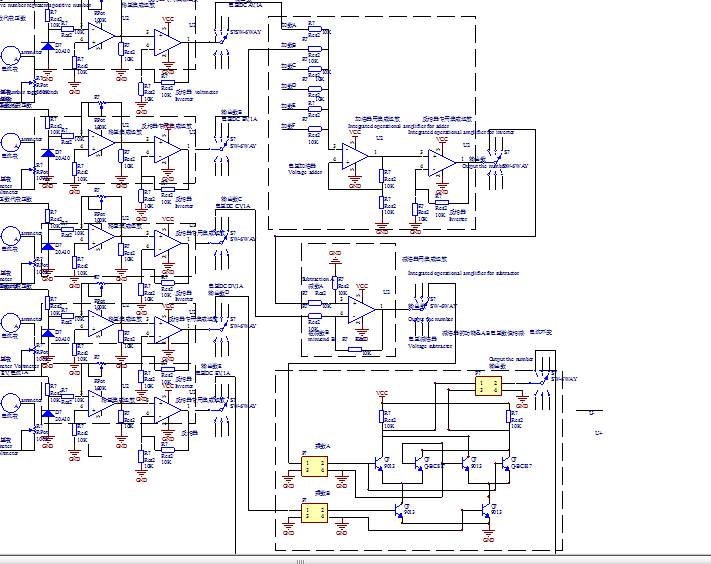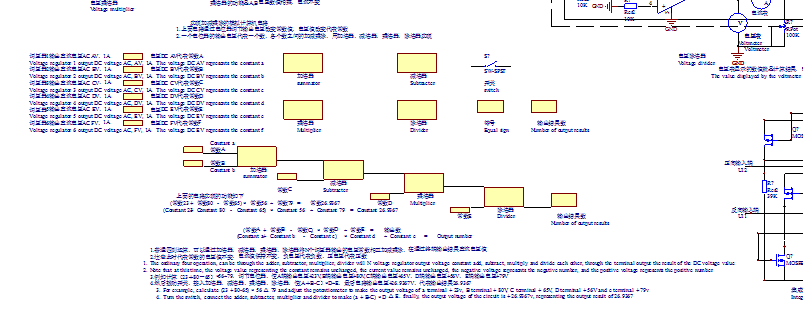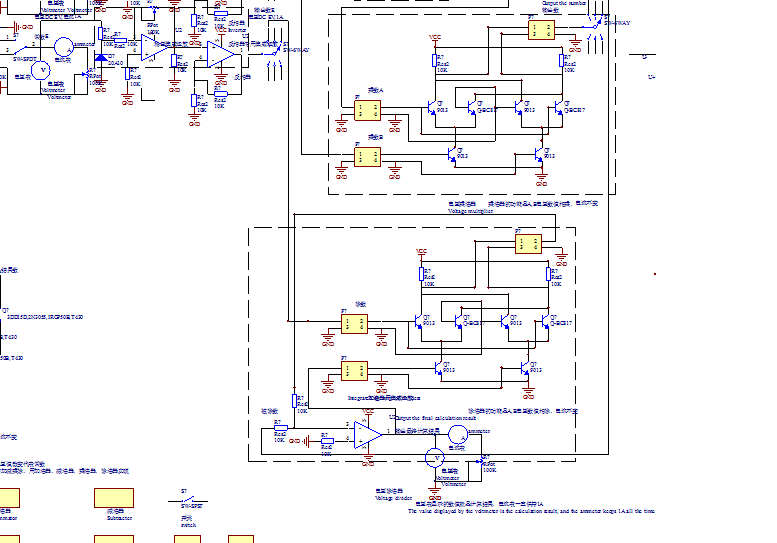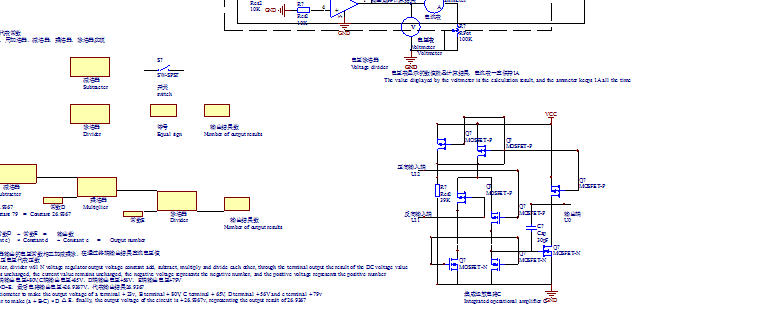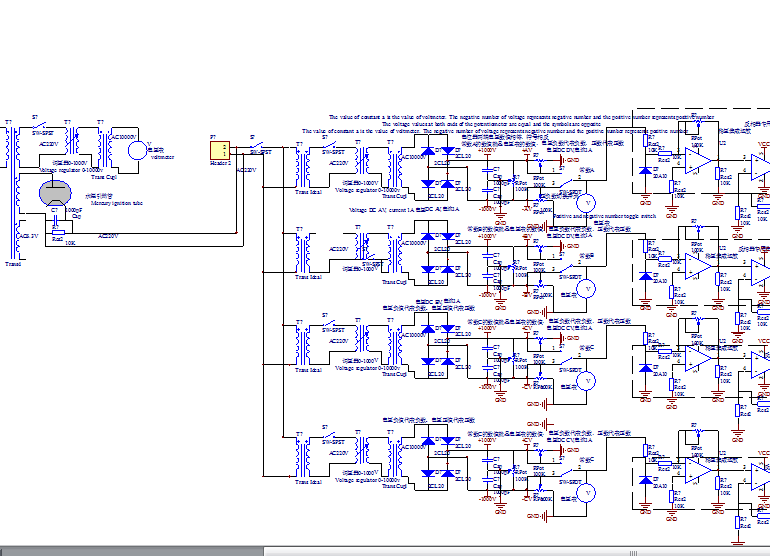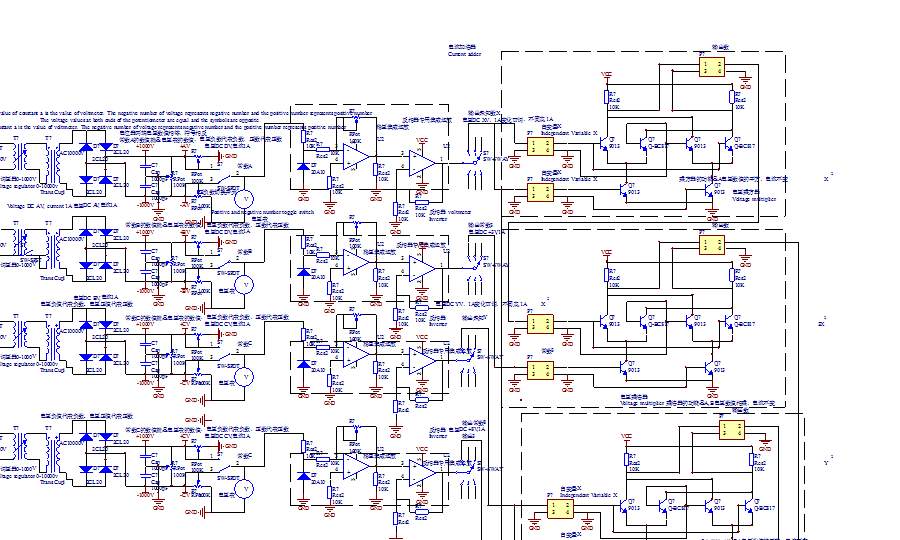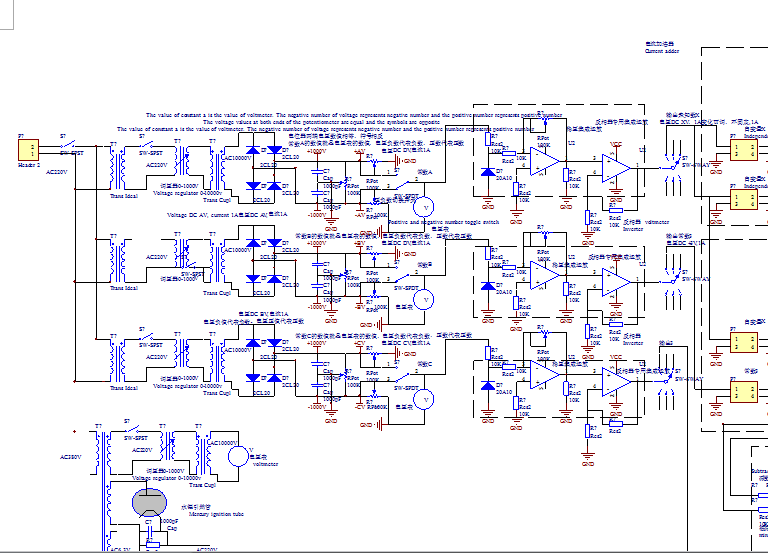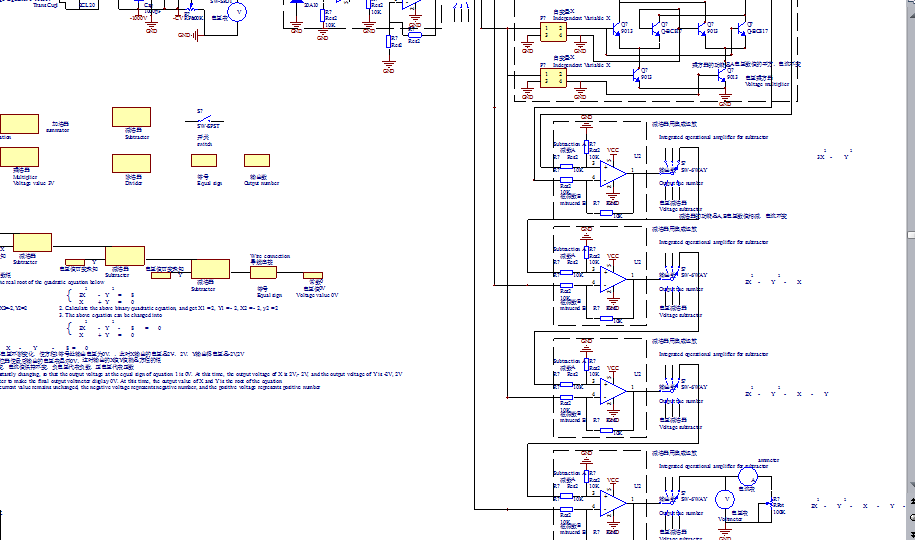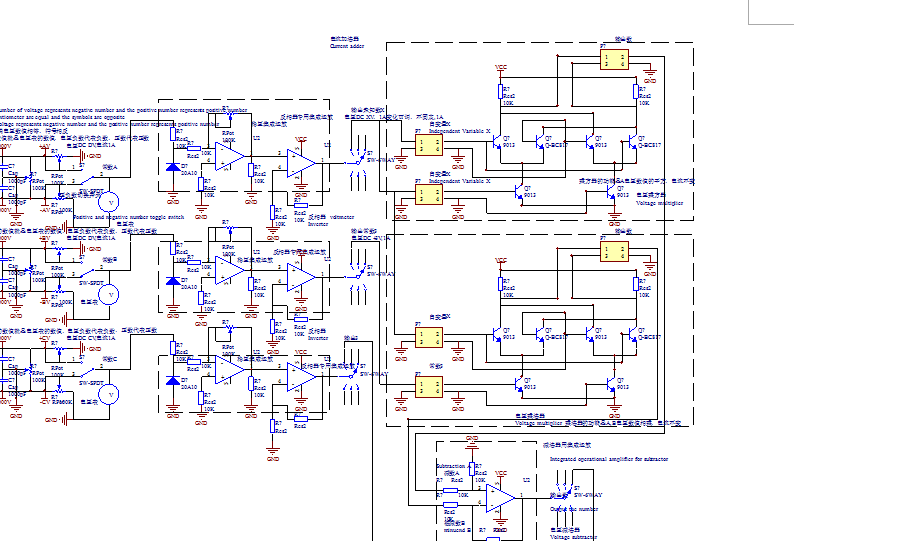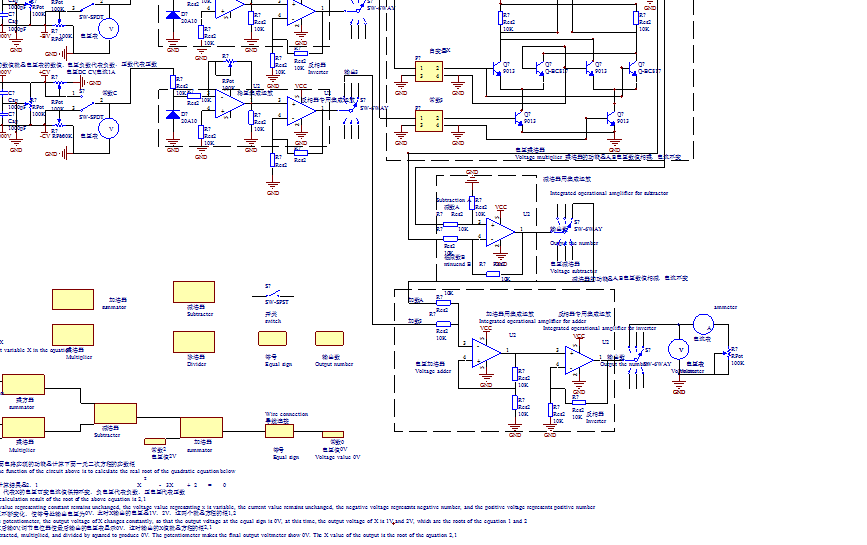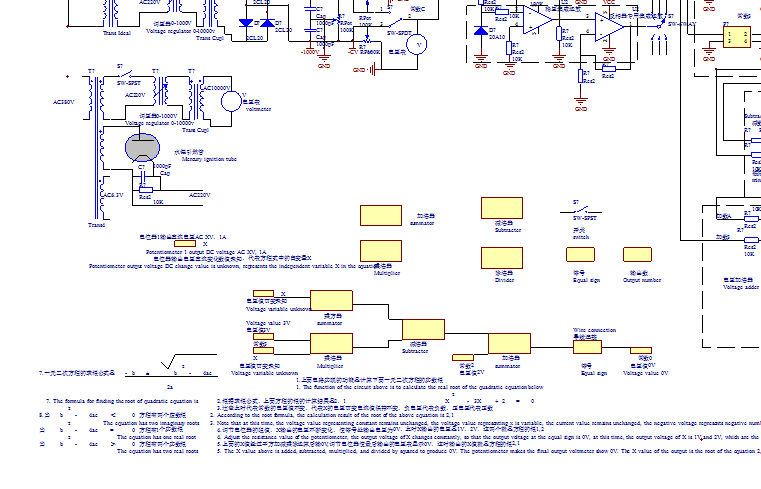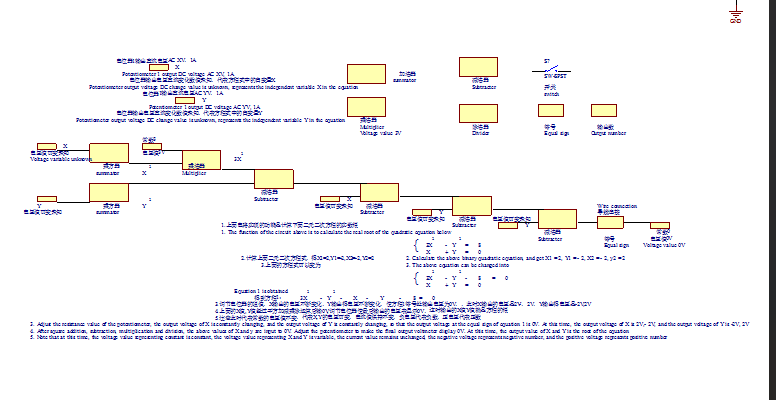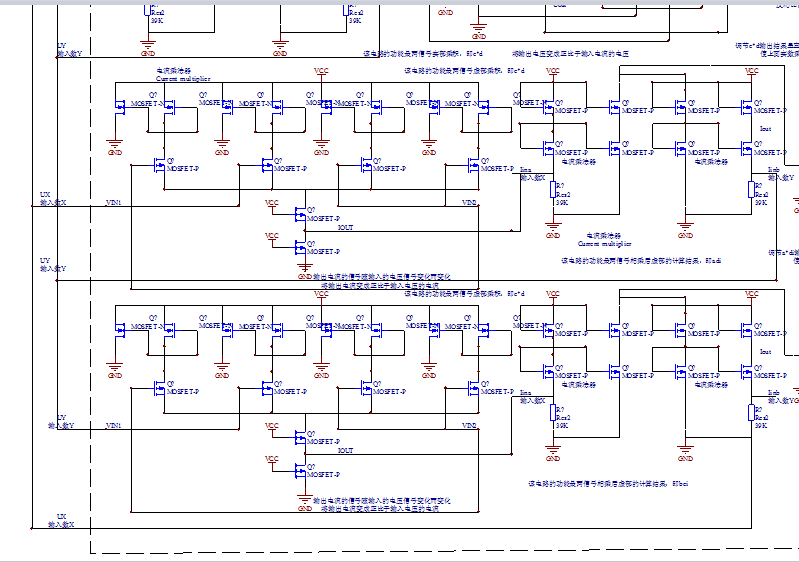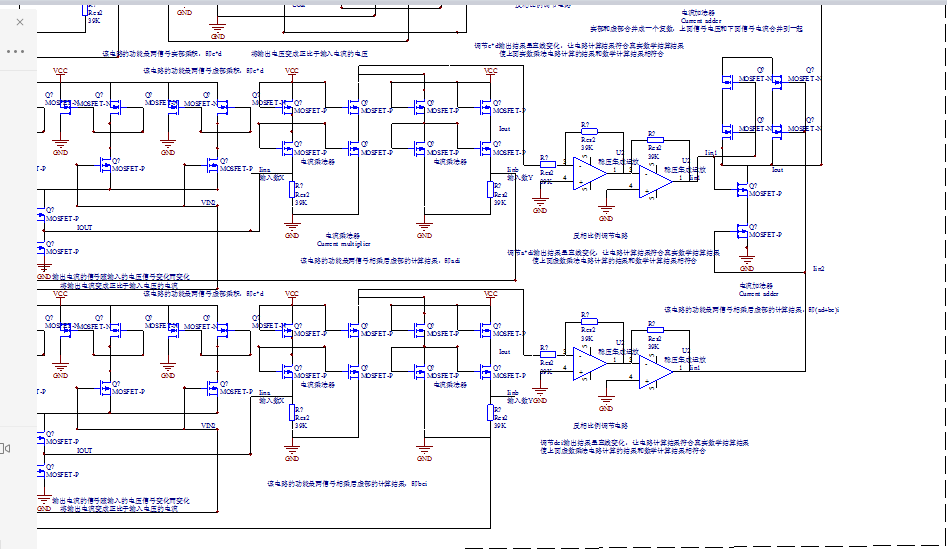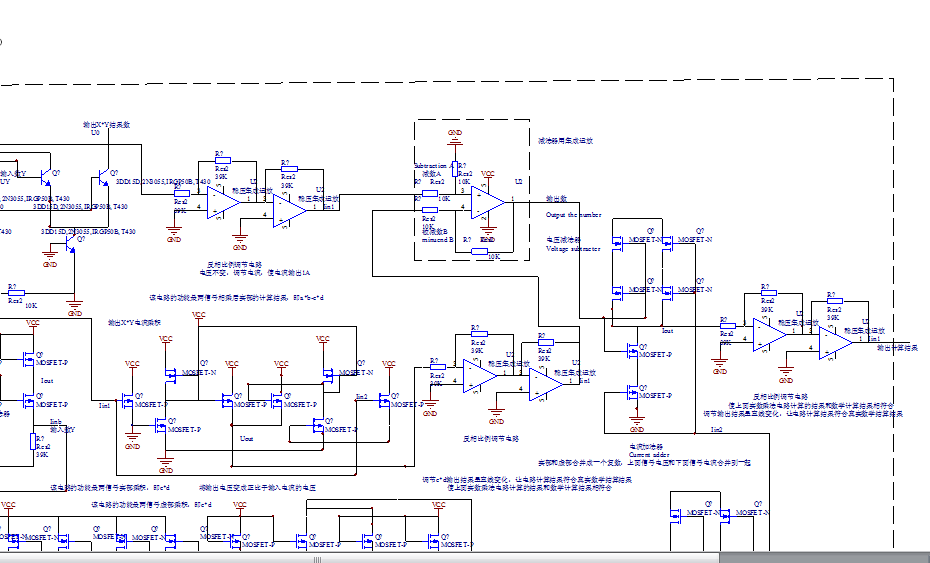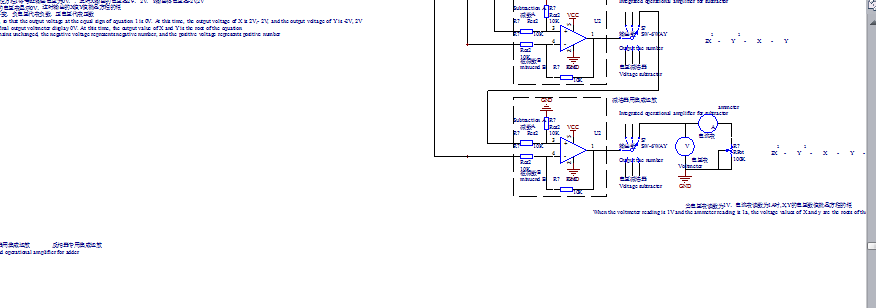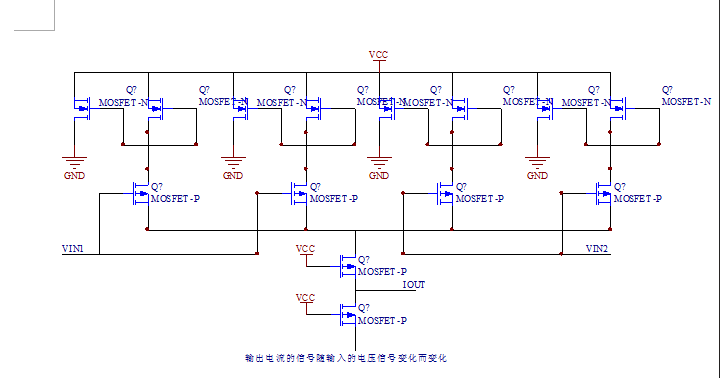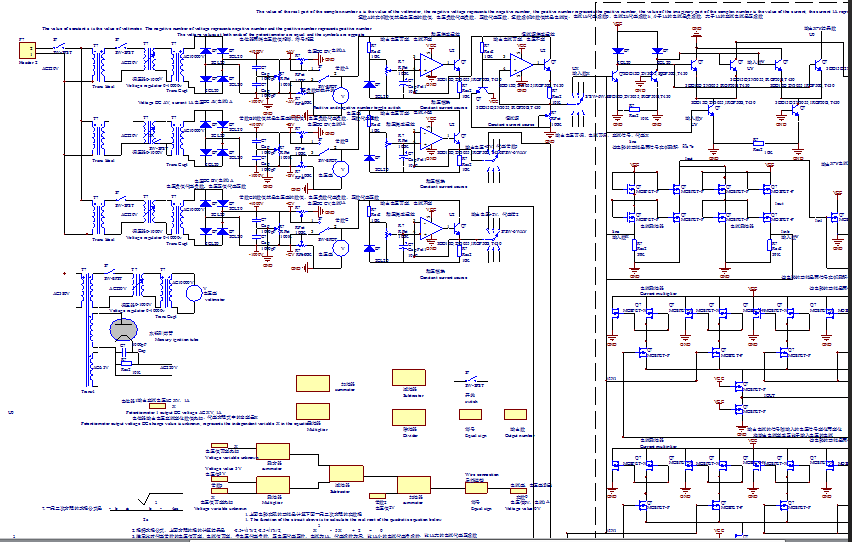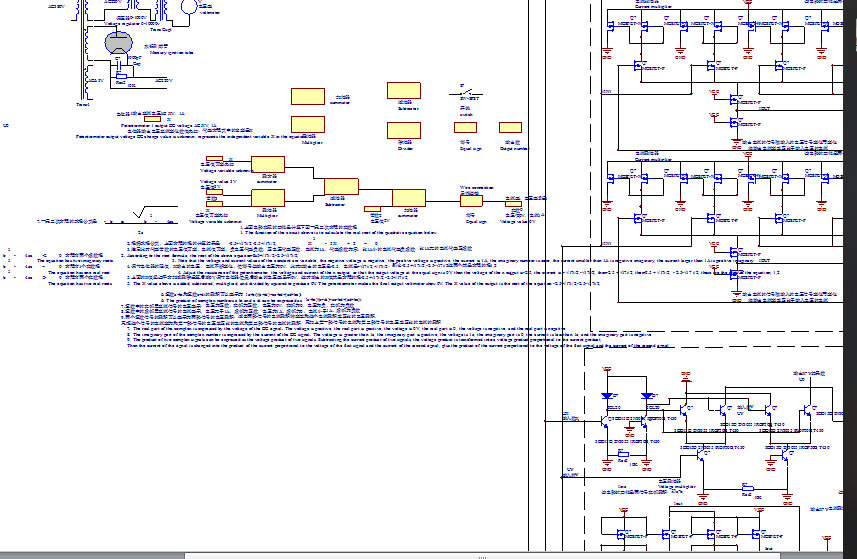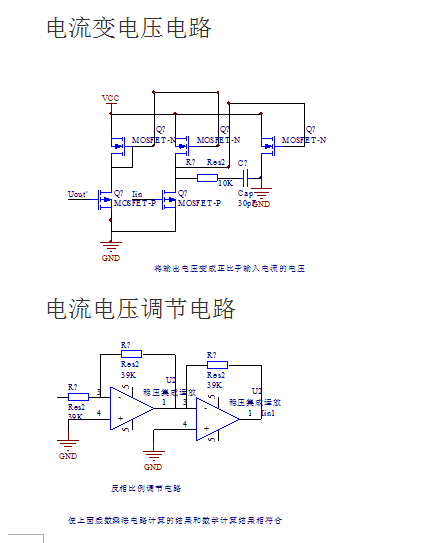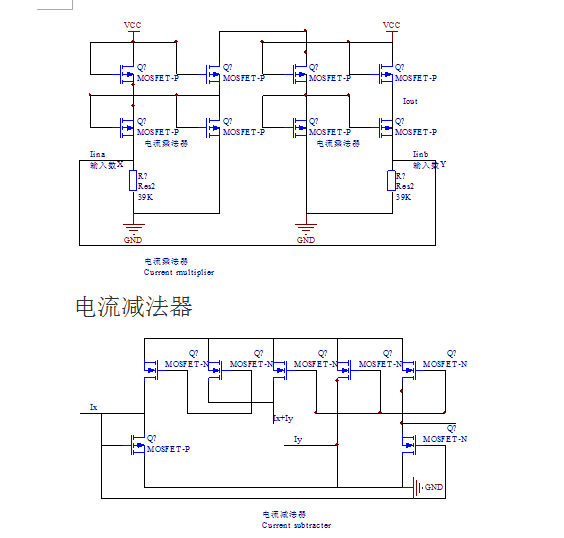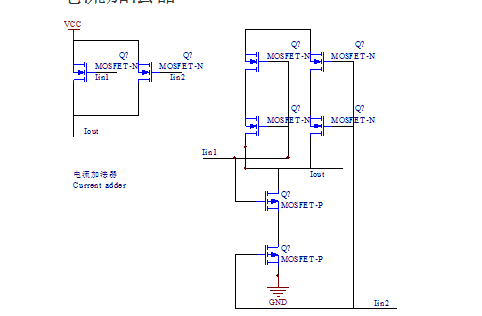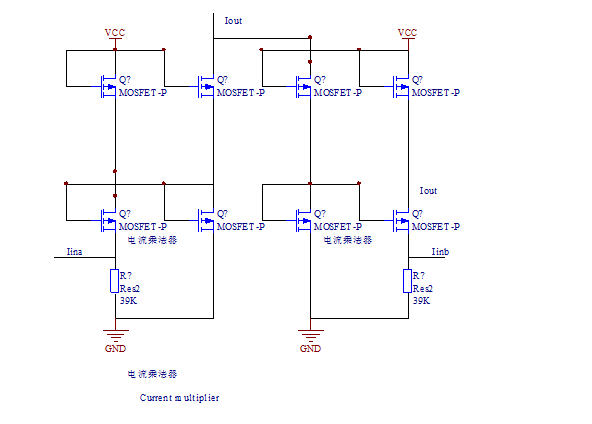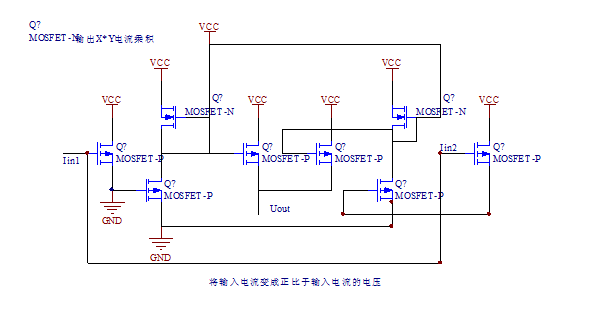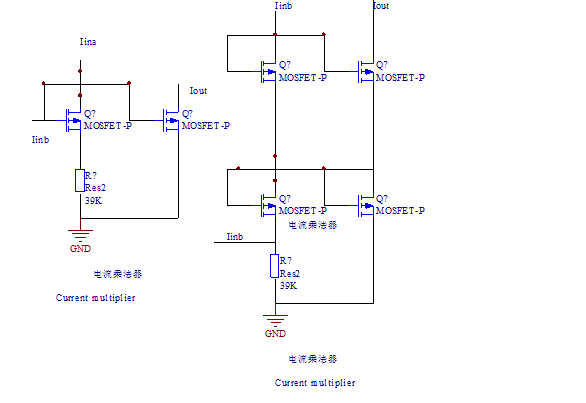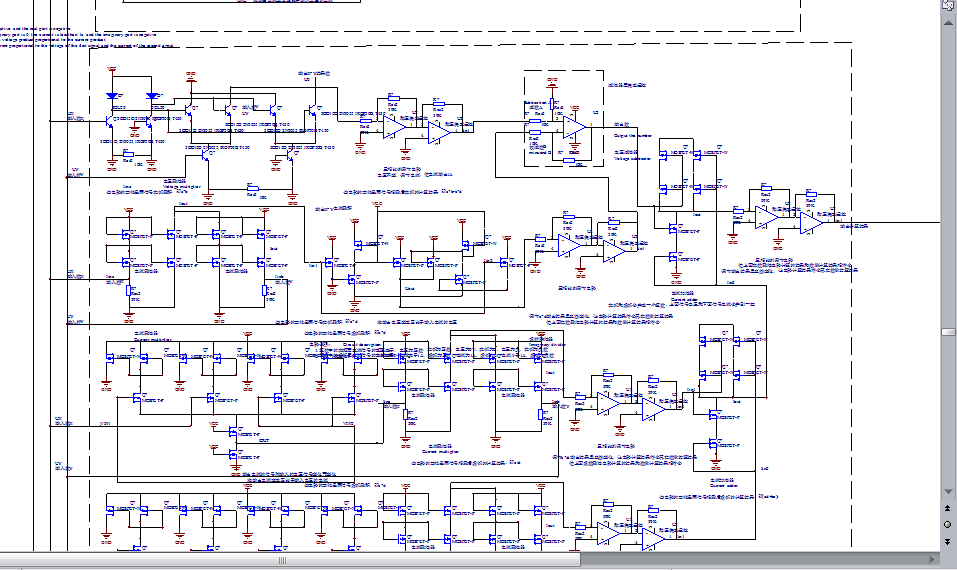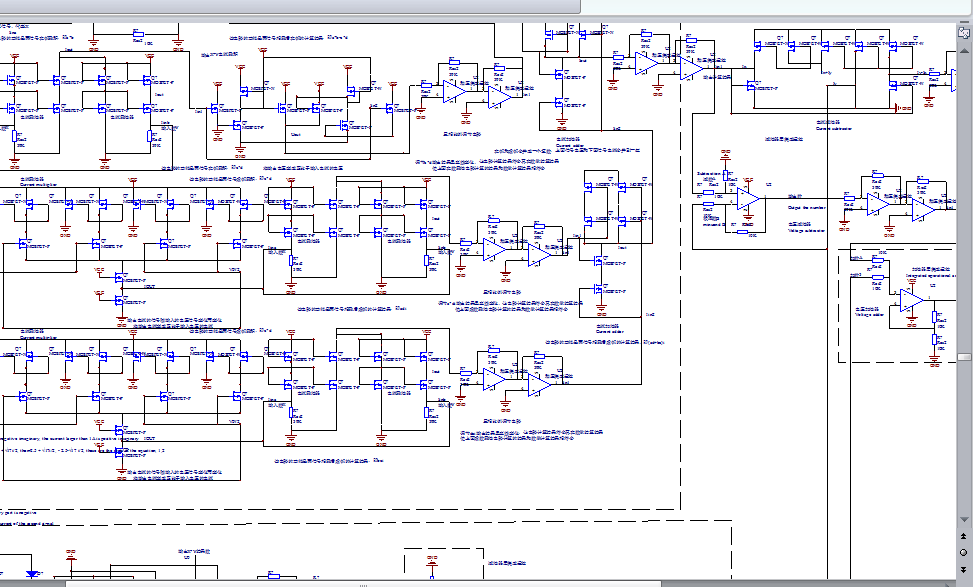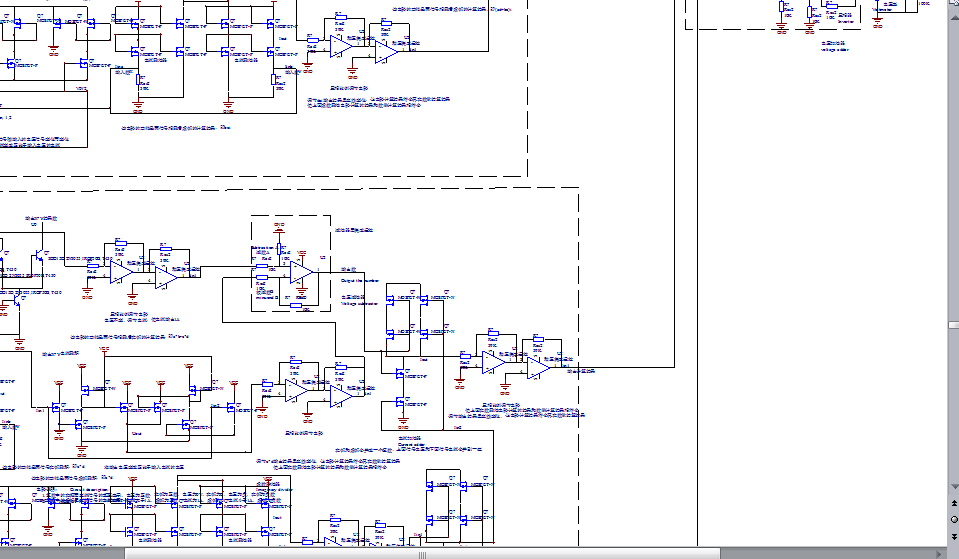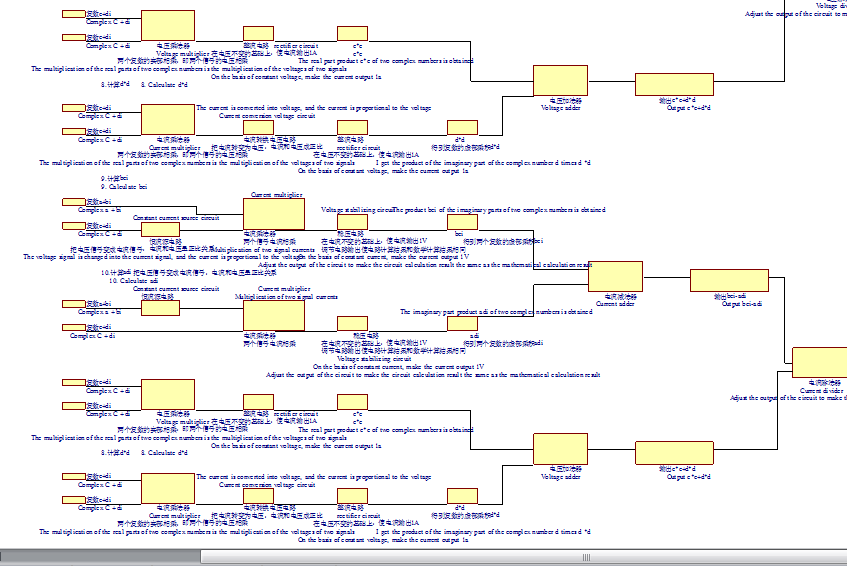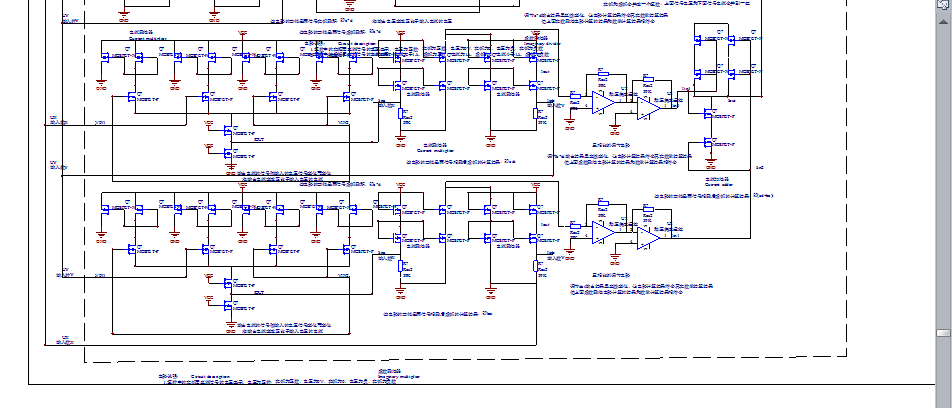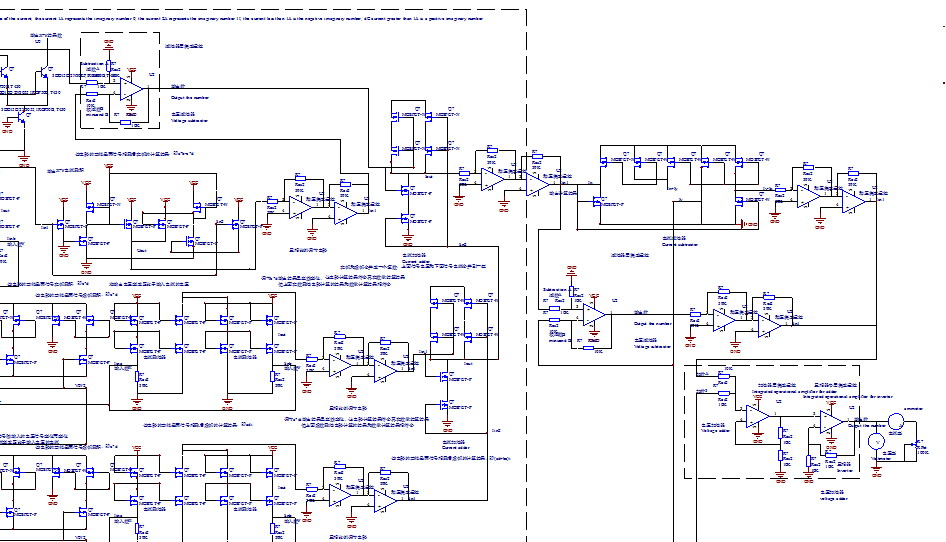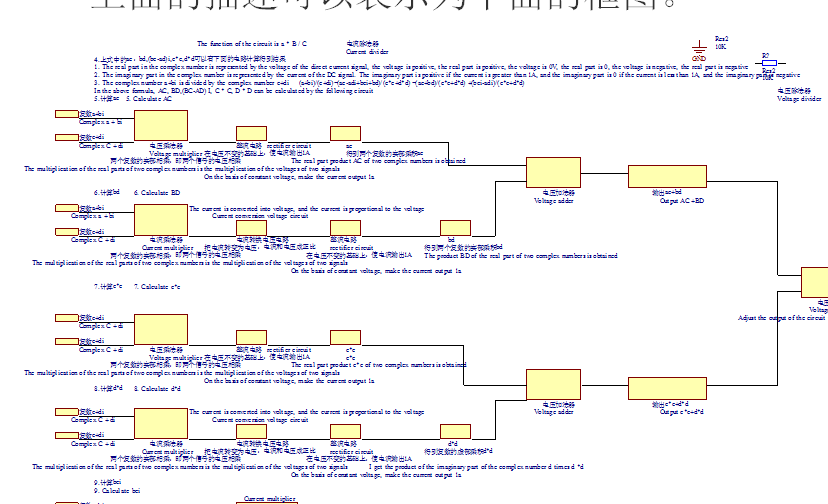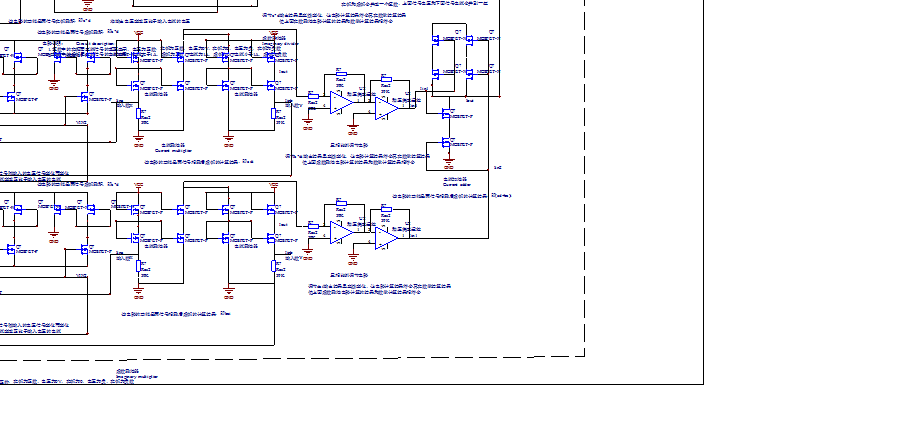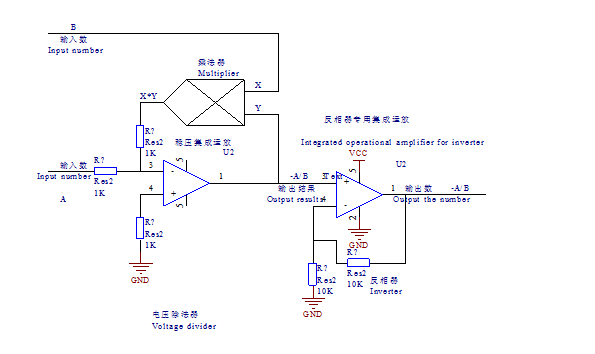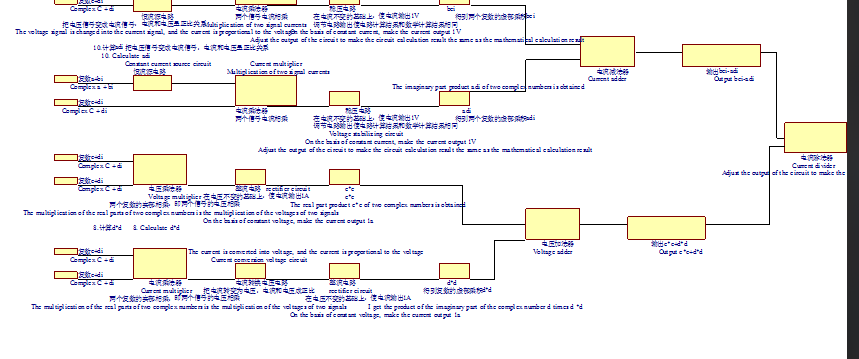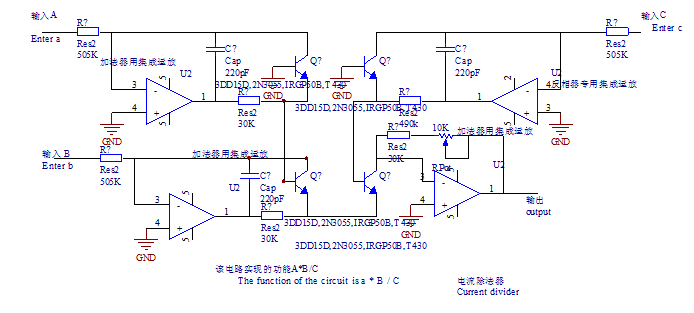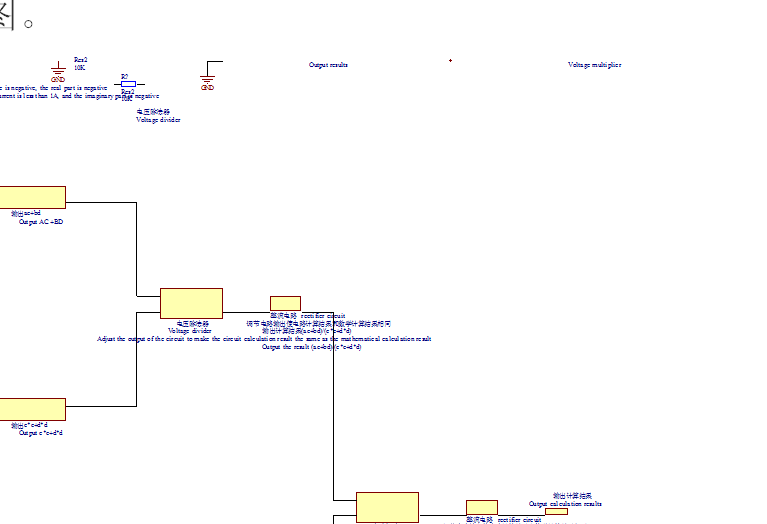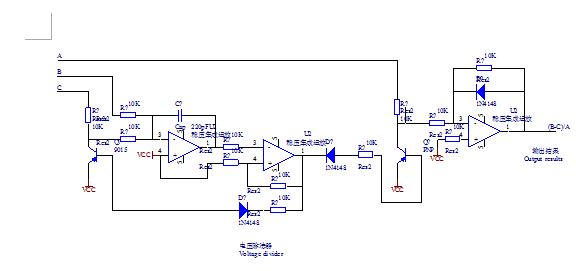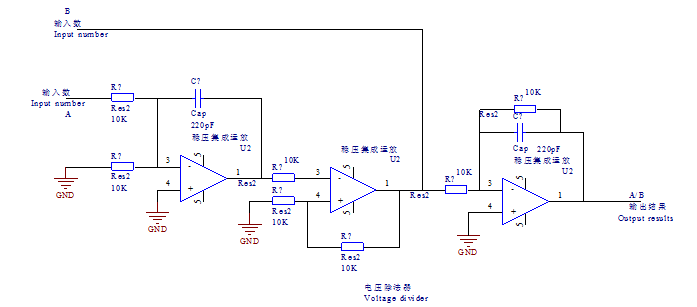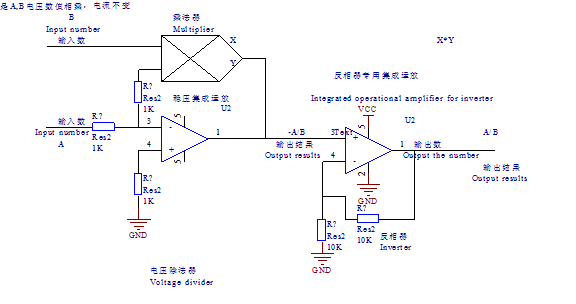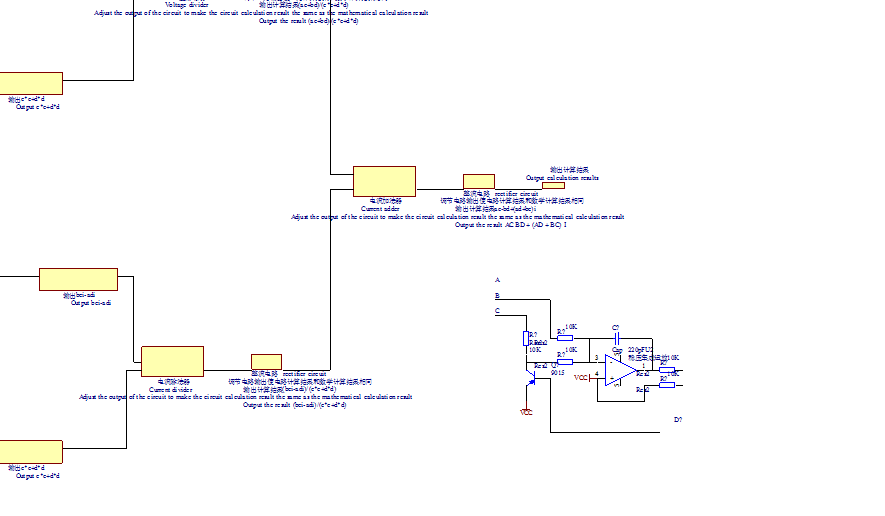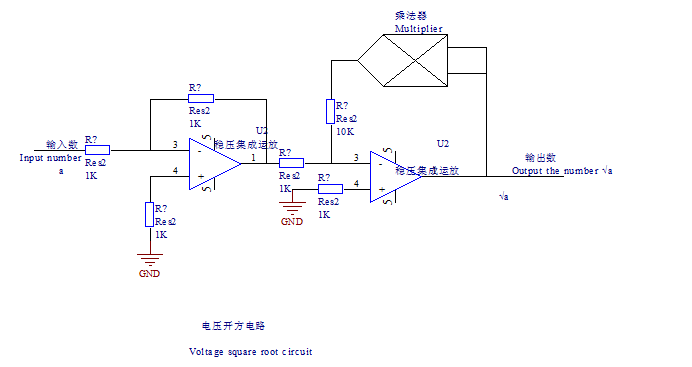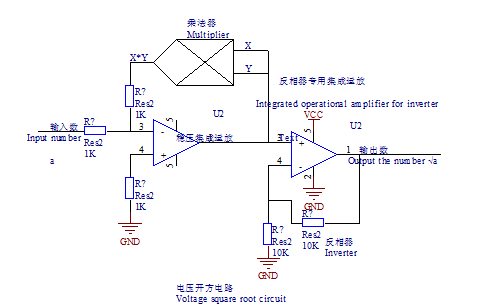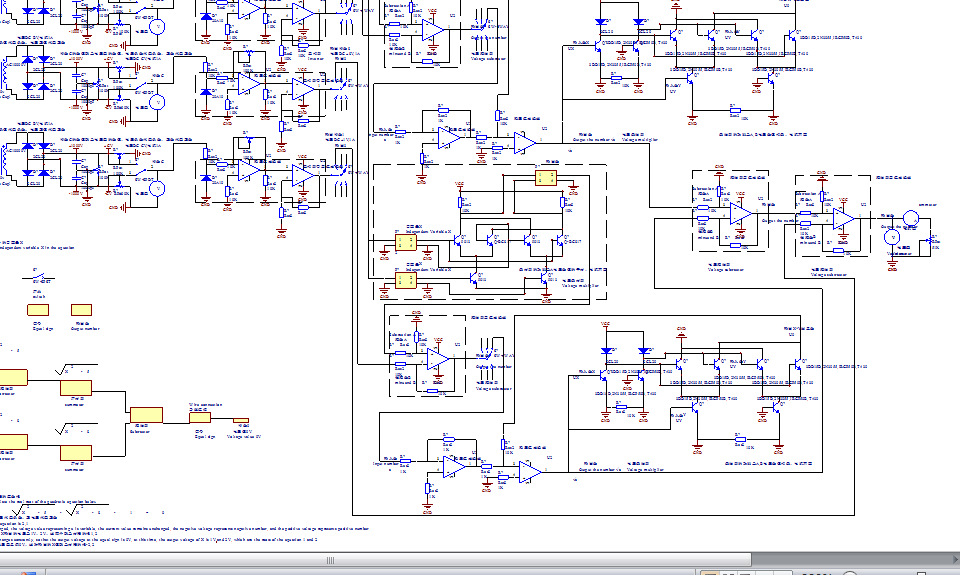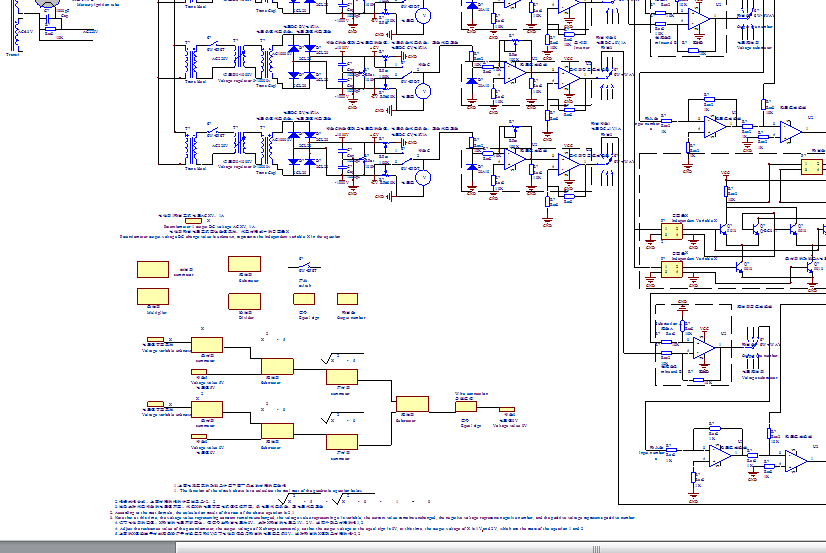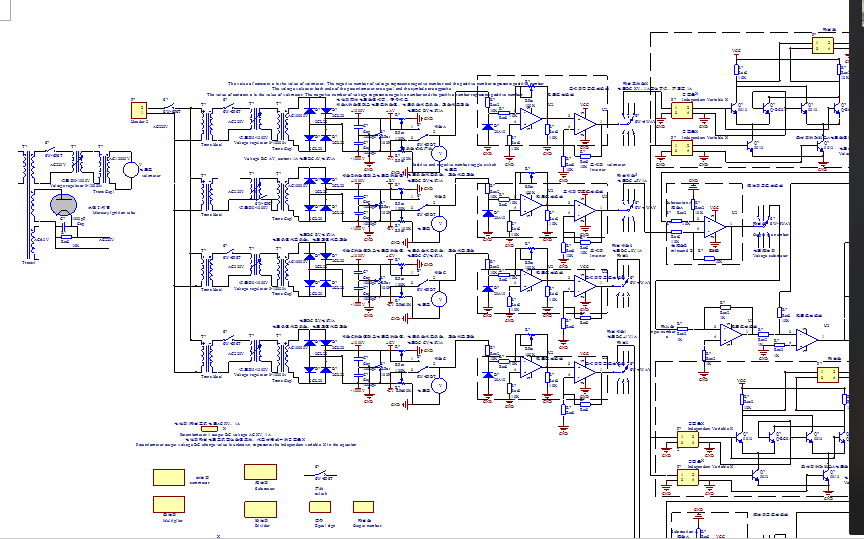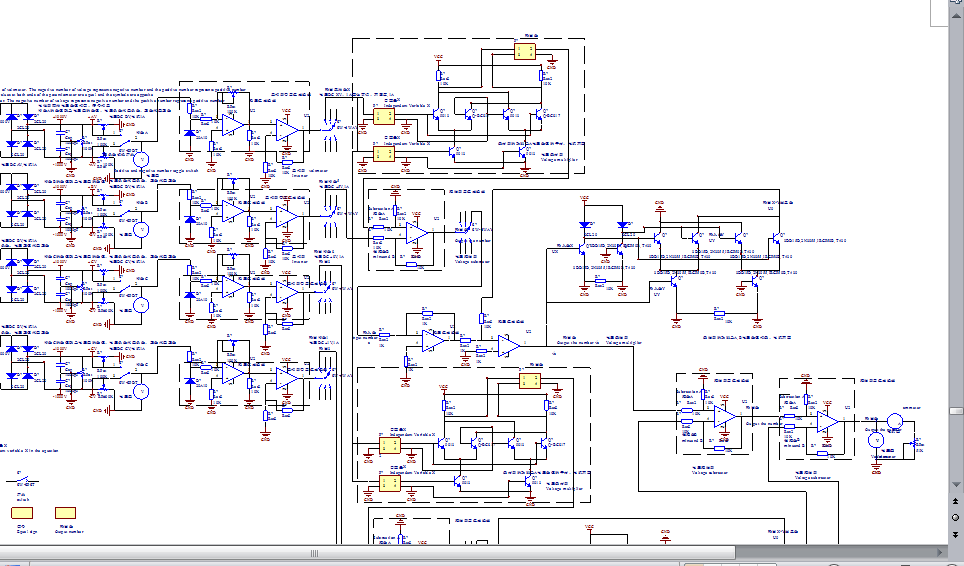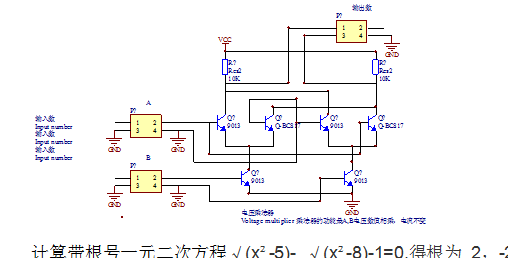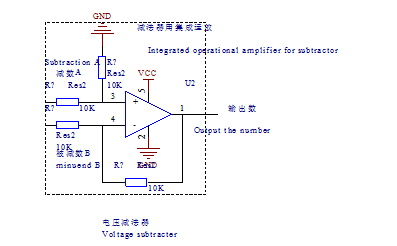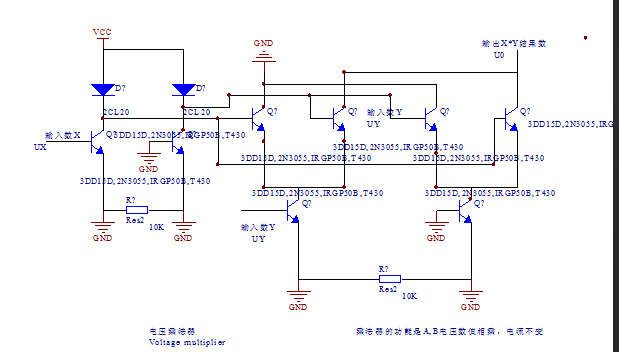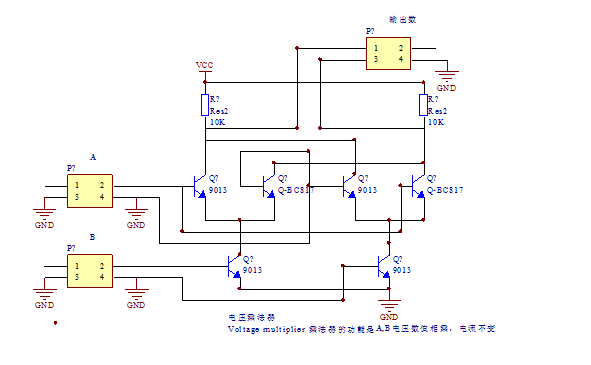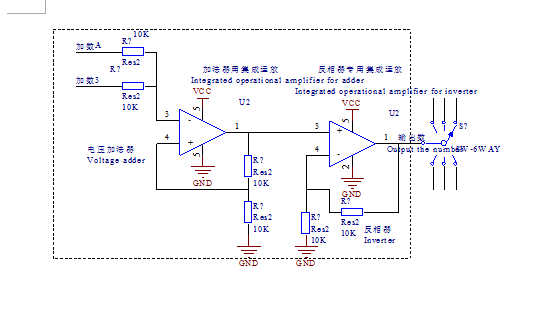展开全文• 给出抽象数据类型复数的定义，并应用其写出求一元二次方程的算法，最后编写程序实现输入一个一元二次方程的三个复数系数，然后输出方程的两个得数根。 注：要求编写的程序是键状的，即能够检测出用户输入的错误，...c++ java
• 实数复数解均可 #include<iostream> using namespace std; void Calculate(double a, double b, double c) { double m = b * b - 4 * a * c; if (m == 0) { cout << "该方程只有一个"<...
实数解与复数解均可
#include<iostream>

using namespace std;

void Calculate(double a, double b, double c)
{
double m = b * b - 4 * a * c;
if (m == 0)
{
cout << "该方程只有一个解"<<endl;
cout <<"x=" << -a * 2 / b;
}
if (m > 0)
{
cout << "该方程有两个不同的实根" << endl;
double m0 = (-b + sqrt(m)) / (2 * a);
double n0 = (-b - sqrt(m)) / (2 * a);
cout << "x1=" << m0 << "  x2=" << n0 ;
}
if (m < 0)
{
cout << "该方程有两个不同的复数根" << endl;
if (b != 0)
{

double m1 = -b  / (2 * a);
double m2 = sqrt(-m) / (2 * a);
double n1 = -b  / (2 * a);
double n2 = - sqrt(-m) / (2 * a);
cout << "x1=" << m1 << (m2 > 0 ? "+" : "-") << fabs(m2) << "i" << "  x2=" << n1 << (n2 > 0 ? "+" : "-") << fabs(n2) << "i";
}
else
{
double m3 = sqrt(-m) / (2 * a);
double n3 = -sqrt(-m) / (2 * a);
cout << "x1=" << m3 << "i   x2=" << n3 << "i";
}

}
}

int main()
{
double a, b, c;
cout << "本程序用于求解一元二次方程（a*x_2+b*x+c=0的根." << endl << "请输入对应的系数:";
cin >> a >> b >> c;
Calculate(a, b, c);

system("pause");
return 0;
}

展开全文c++
• 用vb编写的一元二次方程求解程序。可自动判断的个数，并自动求出方程。 初中数学教师在制作课件时可以把本程序链接到PPT中，增加课件的功能，也可以独立运行单独实现快速求解一元二次方程
• 一元二次方程 本题是if语句的灵活使用，具体有4步： （1）a=0,不是一元二次方程。 （2）b*b-4*a*c=0,有两个相同的实根。 （3）b*b-4*a*c>0.有两个不等实根。 （4）b*b-4*a*c#include #include #define EXP 0....c语言
• 题目：定义一个函数quadratic(a, b, c)，接收3个参数，返回一元二次方程： ax2 + bx + c = 0 的两个。 import math def quadratic(a,b,c): if not (isinstance(a,(int,float)) and isinstance(b,(int,float))...
• 以c语言编写的关于一元二次方程求解问题的程序，可以求复数解
• 一元二次方程 ax²+bx+c=0 的根包含3种情况(这里仅讨论a!=0的情况) #include<stdio.h> #include<math.h>void ax(int a,int b,int c); //δ>0void bx(int a,int b,int c); //δ=0void cx(int a,int b,...
• 一元二次方程的根 本题目要求一元二次方程的根，结果保留2位小数。 输入格式: 输入在一行中给出3个浮点系数a、b、c，中间用空格分开。 输出格式: 根据系数情况，输出不同结果： 1)如果方程有两个不相等的实数根，...
• 记得第一次学习一元二次方程是在初中的时候。那个时候老师就告诉我们，一元二次方程一般有两个根，一元n次方程则一般有n个根。后来在课外书上了解到，这个结论最早是由数学王子高斯严格证明出来的。当时我就有一个...
• 主要介绍了Python实现求解一元二次方程的方法,涉及Python基于math包进行数值运算相关操作技巧,需要的朋友可以参考下
• 综合上述笔记，自己写的练习题。考虑了特殊字符转换 float 的情况：import cmathdef mx(a,b,c):if a==0:if...= 0print('一次方程 {} * x + {} = 0 的解为：\n x = {:.3f}'.format(b,c,-c/b))returnelse: #a != 0prin...
• 一元二次方程的根本题目要求一元二次方程的根，结果保留2位小数。输入格式:输入在一行中给出3个浮点系数a、b、c，中间用空格分开。输出格式:根据系数情况，输出不同结果：1)如果方程有两个不相等的实数根，则每行...C语言 PTA题目
• 复数范围内求解一元二次方程组，可以用作C++的入门练习参考。
• 一元二次方程ax^2+bx+c=0的。a,b,c任意实数。 Input 输入数据有一行，包括a b c的值。 Output 按以下格式输出方程的根x1和x2。x1和x2之间有一个空格。 x1 x2 （1）如果x1和x2实根，则以x1>=x2输出。 （2...
• 这是一个用Java写的分形程序，包括雪花图形、Sierpinski地毯还有一元二次方程的实数根和复数跟的空间四个图形。
• 标签：basicscanf二次int题目语言laninclude程序本题目要求一元二次方程的根，结果保留2位小数。输入格式:输入在一行中给出3个浮点系数a、b、c，中间用空格分开。输出格式:根据系数情况，输出不同结果：1)如果方程有...
• #include <stdio.h> #include <math.h> int main() { double a, // 二次项系数 b, // 一次项系数 c; // 常数项 double delta; // 第一步：输入必要的数据；输入一元二次方程各项系数的...
• #include &lt;stdio.h&gt; int panduan(int a,int b,int c) { int m; m=b*b-4*a*c; return m; } int main() { int flag; int ture,v; int a,b,c; int x1,x2; scanf("%d%d%d"...a...
• C++ 实例 - 求一元二次方程的根 实现方法相当简单 就是最基础的C语言程序 以下是菜鸟教程给的答案—— #include <iostream> #include <cmath> using namespace std; int main() { float a, b, c, ...c++...